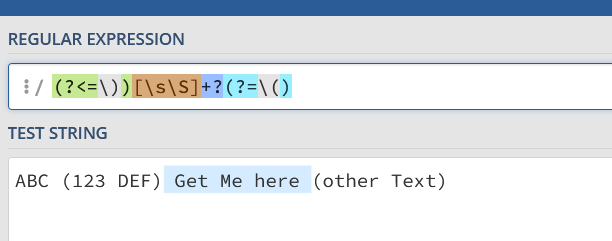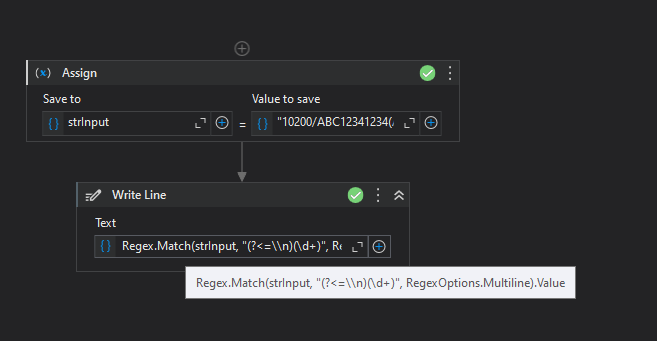# Value between brackets

Hi All,

I want to extract value between the brackets using regular expression. Kindly help.Need value 11000.

Regards
Anand

Hi @anand.t

can you give an example what data to be extracted.

For example:

10200/ABC10831100(ABC Bank - 10831100)\n11000(Bank Data )

I need only 11000.[CheatSheet] - System.Text.RegularExpressions | RegEx - News / Tutorials - UiPath Community Forum

`(?<=\))[\s\S]+?(?=\()`
then we trim the value

another method

(?<=\n)\d+(?=()

regards

Hi @anand.t

Give a try with``````Regex.Match(strInput, "(?<=\\n)(\d+)", RegexOptions.Multiline).Value
``````

Regards

Hi @anand.t How about the below one

``````(?<=\(.*\)\\n).*(?=\(.*\))
``````

.NET Regex Tester - Regex Storm

Thanks All